# Trigonometric Equations Section 5 5 Objectives Solve trigonometric

• Slides: 34Trigonometric Equations Section 5. 5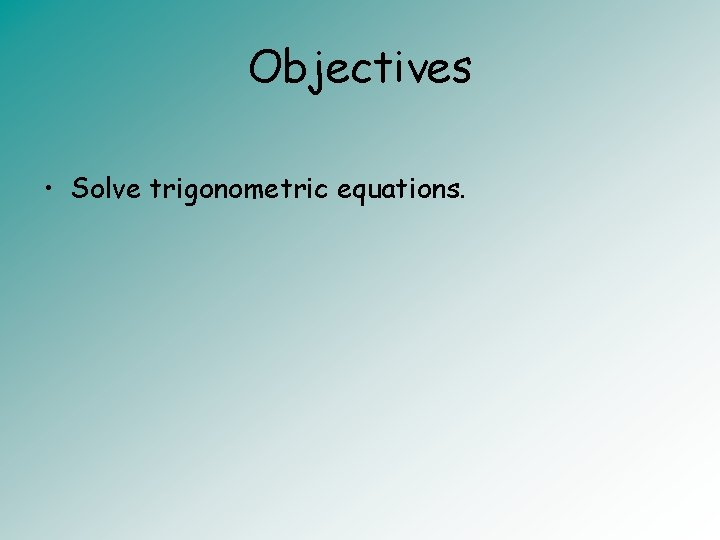Objectives • Solve trigonometric equations.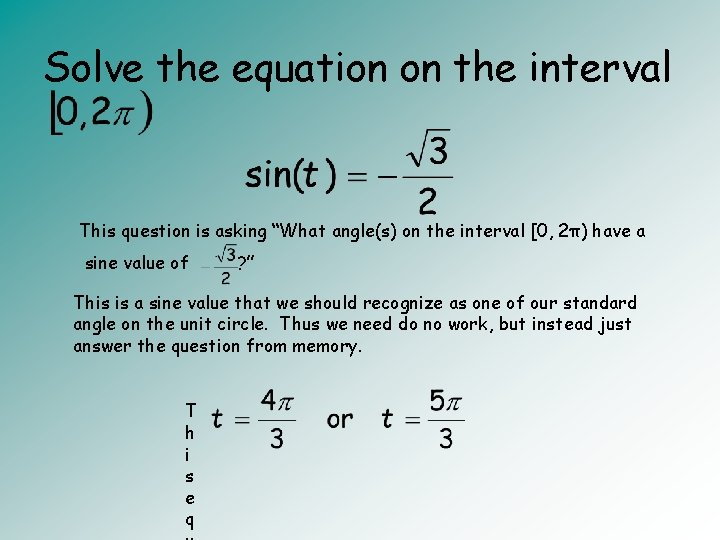Solve the equation on the interval This question is asking “What angle(s) on the interval [0, 2π) have a sine value of ? ” This is a sine value that we should recognize as one of our standard angle on the unit circle. Thus we need do no work, but instead just answer the question from memory. T h i s e q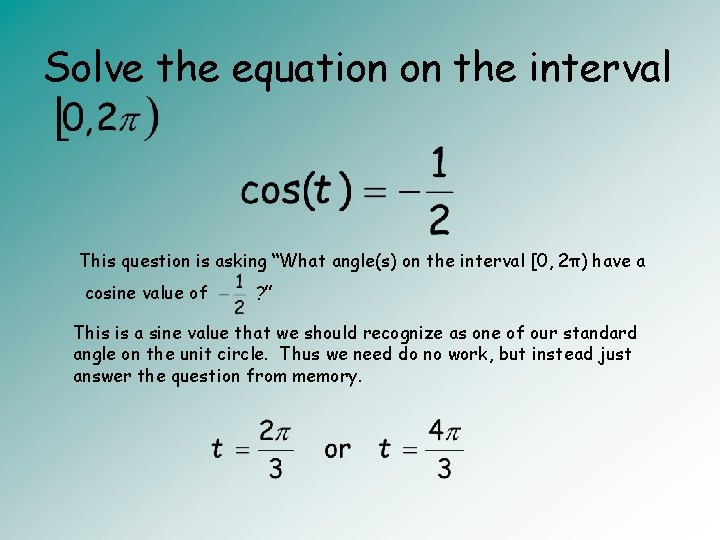Solve the equation on the interval This question is asking “What angle(s) on the interval [0, 2π) have a cosine value of ? ” This is a sine value that we should recognize as one of our standard angle on the unit circle. Thus we need do no work, but instead just answer the question from memory.Solve the equation on the interval Although we recognize the ½ as a value we know, since the sine function is squared, we first must take the square root of both sides of the equation. continued on next slide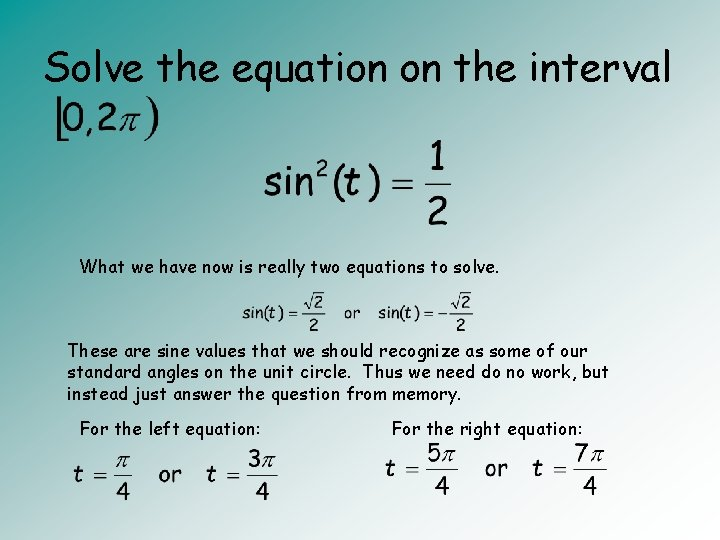Solve the equation on the interval What we have now is really two equations to solve. These are sine values that we should recognize as some of our standard angles on the unit circle. Thus we need do no work, but instead just answer the question from memory. For the left equation: For the right equation:Solve the equation on the interval For this problem, we must do some algebraic work to get to an equation like the previous ones. Here we need to factor. This equation can be factored by grouping. The grouping can be seen here with the red and blue boxes. The part surrounded by the red box has a cos(t) that can be factored out of both pieces. Now you should notice that the part in the square brackets and the part in the blue box are the same. This means that we can factor this part out. continued on next slide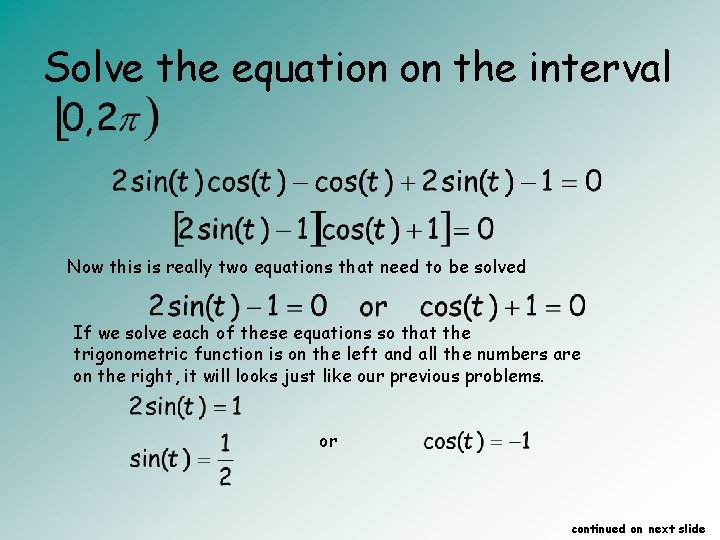Solve the equation on the interval Now this is really two equations that need to be solved If we solve each of these equations so that the trigonometric function is on the left and all the numbers are on the right, it will looks just like our previous problems. or continued on next slideSolve the equation on the interval Each of these equations, we should know the answers to. or orSolve the equation on the interval In order to do this problem, we need to angles to be the same. The angle in the sine function is 2 x. The angle in the cosine function is x. Since we have an identity for the double angle of a sine function, we can replace sin(2 x) with the identity. This will give us the same angles in all of the trigonometric functions. Now we can factor a cos(x) out of each piece to get: continued on next slideSolve the equation on the interval In order to do this problem, we need to angles to be the same. The angle in the sine function is 2 x. The angle in the cosine function is x. Since we have an identity for the double angle of a sine function, we can replace sin(2 x) with the identity. This will give us the same angles in all of the trigonometric functions. Now we can factor a cos(x) out of each piece to get: continued on next slide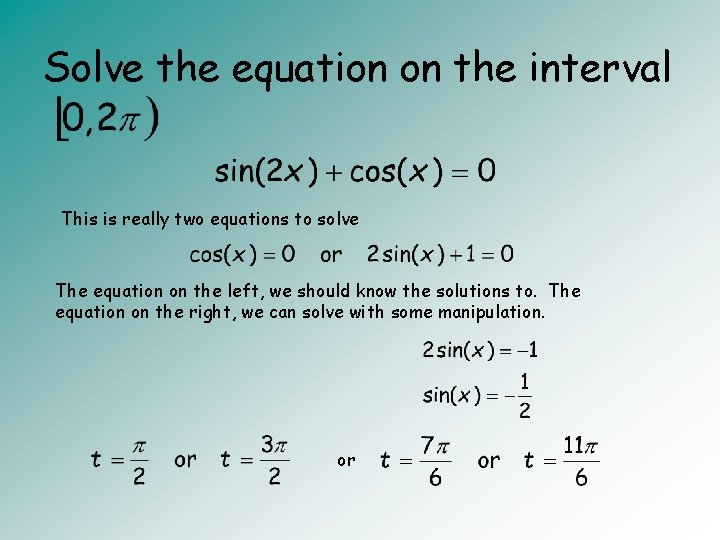Solve the equation on the interval This is really two equations to solve The equation on the left, we should know the solutions to. The equation on the right, we can solve with some manipulation. orSolve the equation on the interval Our first step in the problem will be to take the square root of both sides of the equation. This will give us two equations to solve. or Neither of these is a value that we know from our standard angles. continued on next slide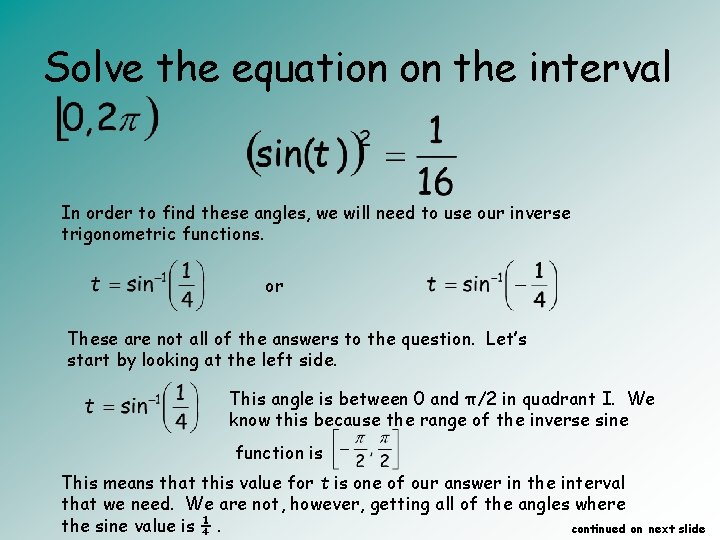Solve the equation on the interval In order to find these angles, we will need to use our inverse trigonometric functions. or These are not all of the answers to the question. Let’s start by looking at the left side. This angle is between 0 and π/2 in quadrant I. We know this because the range of the inverse sine function is This means that this value for t is one of our answer in the interval that we need. We are not, however, getting all of the angles where the sine value is ¼. continued on next slide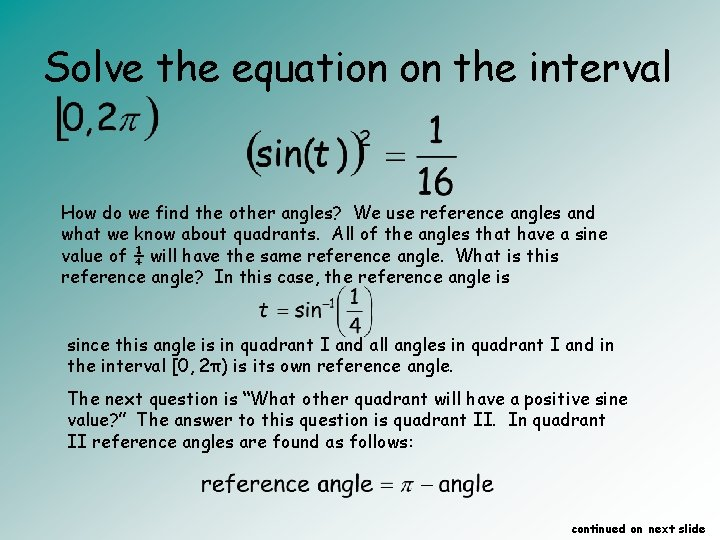Solve the equation on the interval How do we find the other angles? We use reference angles and what we know about quadrants. All of the angles that have a sine value of ¼ will have the same reference angle. What is this reference angle? In this case, the reference angle is since this angle is in quadrant I and all angles in quadrant I and in the interval [0, 2π) is its own reference angle. The next question is “What other quadrant will have a positive sine value? ” The answer to this question is quadrant II. In quadrant II reference angles are found as follows: continued on next slide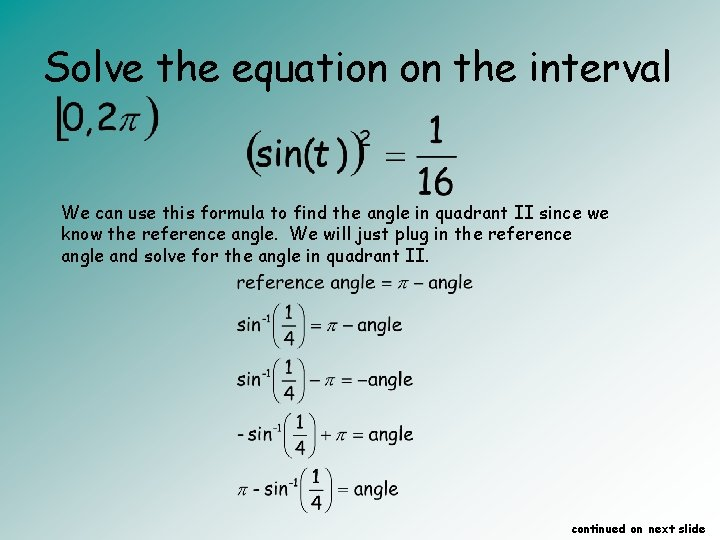Solve the equation on the interval We can use this formula to find the angle in quadrant II since we know the reference angle. We will just plug in the reference angle and solve for the angle in quadrant II. continued on next slide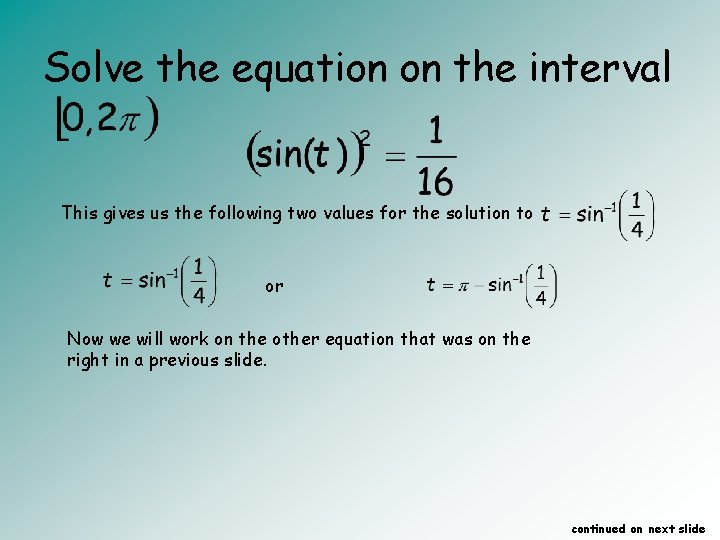Solve the equation on the interval This gives us the following two values for the solution to or Now we will work on the other equation that was on the right in a previous slide. continued on next slide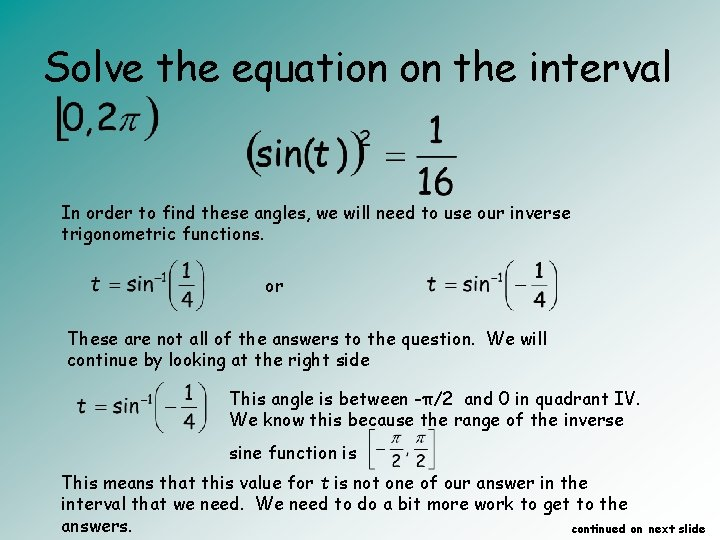Solve the equation on the interval In order to find these angles, we will need to use our inverse trigonometric functions. or These are not all of the answers to the question. We will continue by looking at the right side This angle is between -π/2 and 0 in quadrant IV. We know this because the range of the inverse sine function is This means that this value for t is not one of our answer in the interval that we need. We need to do a bit more work to get to the answers. continued on next slide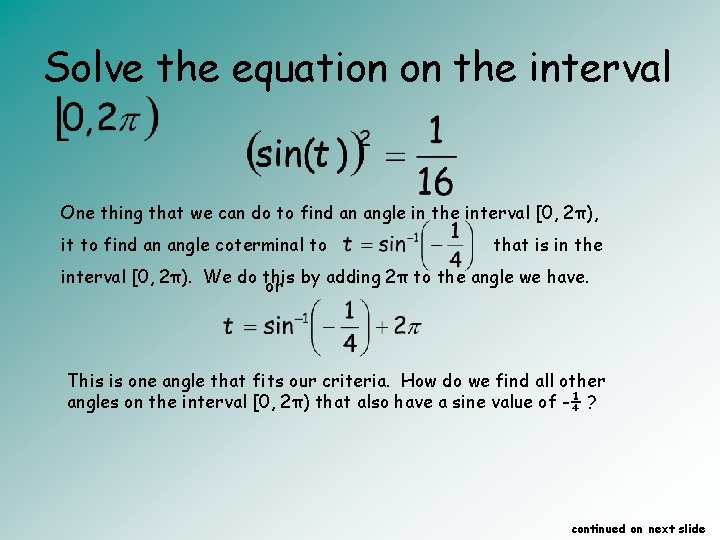Solve the equation on the interval One thing that we can do to find an angle in the interval [0, 2π), it to find an angle coterminal to that is in the interval [0, 2π). We do this or by adding 2π to the angle we have. This is one angle that fits our criteria. How do we find all other angles on the interval [0, 2π) that also have a sine value of -¼ ? continued on next slideSolve the equation on the interval How do we find the other angles? We use reference angles and what we know about quadrants. All of the angles that have a sine value of -¼ will have the same reference angle. What is this reference angle? In this case, we will find the reference angle for the one angle that we have in quadrant IV. continued on next slide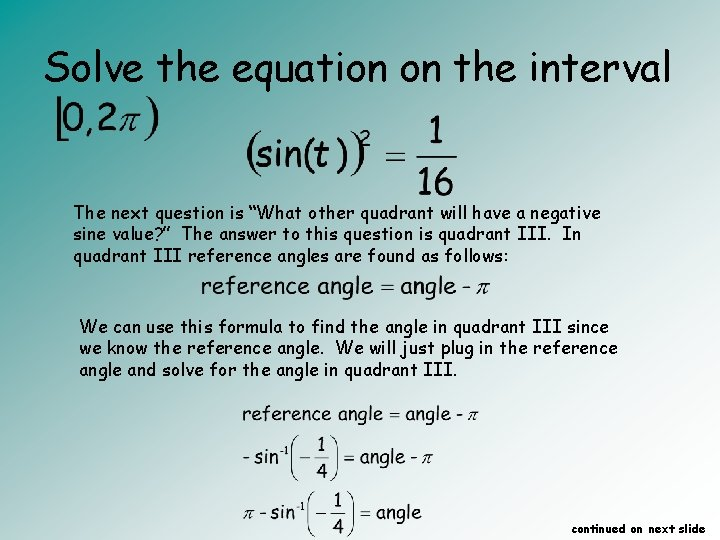Solve the equation on the interval The next question is “What other quadrant will have a negative sine value? ” The answer to this question is quadrant III. In quadrant III reference angles are found as follows: We can use this formula to find the angle in quadrant III since we know the reference angle. We will just plug in the reference angle and solve for the angle in quadrant III. continued on next slide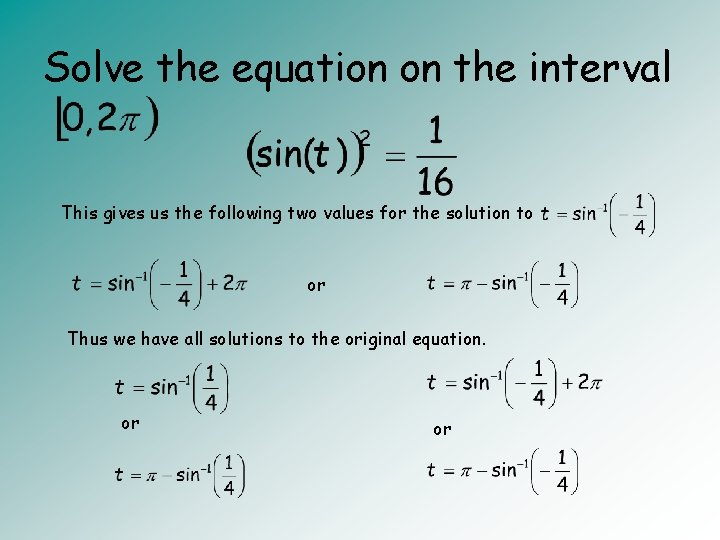Solve the equation on the interval This gives us the following two values for the solution to or Thus we have all solutions to the original equation. or or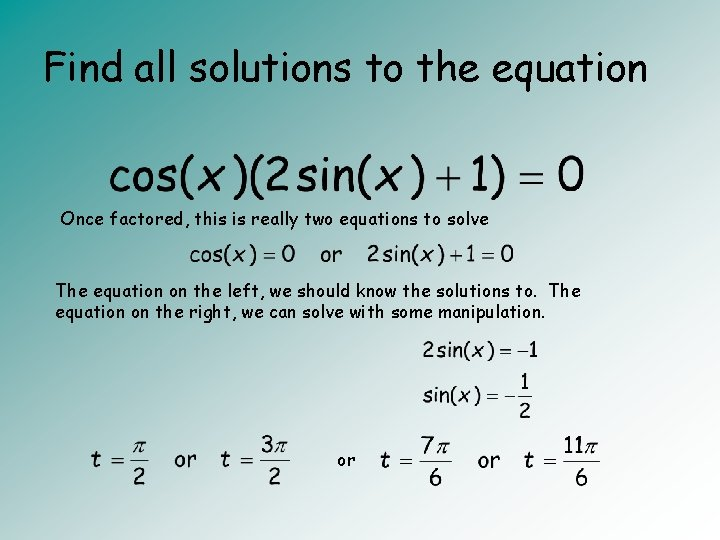Find all solutions to the equation Once factored, this is really two equations to solve The equation on the left, we should know the solutions to. The equation on the right, we can solve with some manipulation. or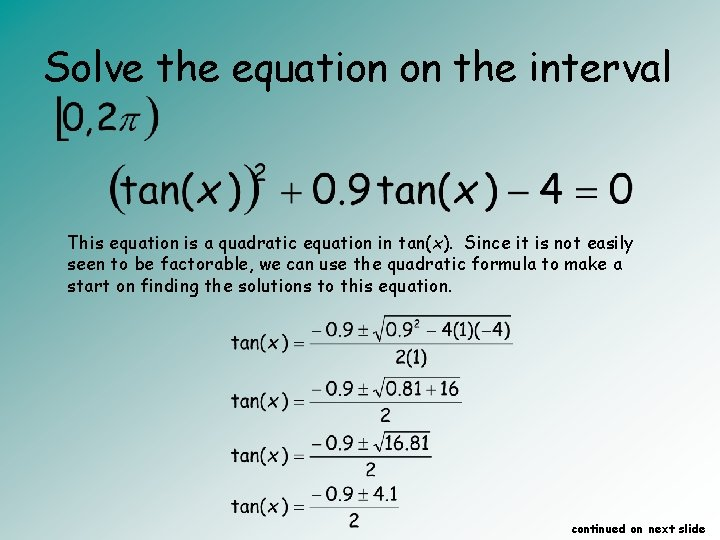Solve the equation on the interval This equation is a quadratic equation in tan(x). Since it is not easily seen to be factorable, we can use the quadratic formula to make a start on finding the solutions to this equation. continued on next slideSolve the equation on the interval This is really two equations to solve. We are going to go through the same process that we did with the previous problem to find the answers. continued on next slideSolve the equation on the interval In order to find these angles, we will need to use our inverse trigonometric functions. or These are not all of the answers to the question. Let’s start by looking at the left side. This angle is between 0 and π/2 in quadrant I. We know this because the range of the inverse tangent function is This means that this value for t is one of our answer in the interval that we need. We are not, however, getting all of the angles where the tangent value is 1. 6. continued on next slide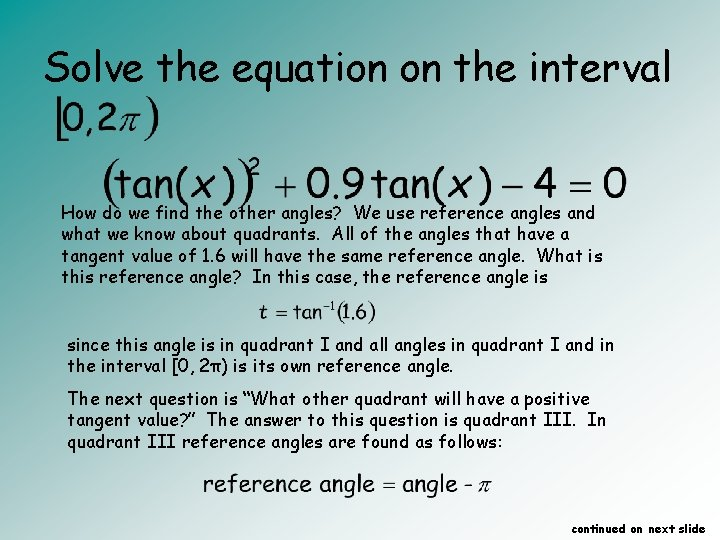Solve the equation on the interval How do we find the other angles? We use reference angles and what we know about quadrants. All of the angles that have a tangent value of 1. 6 will have the same reference angle. What is this reference angle? In this case, the reference angle is since this angle is in quadrant I and all angles in quadrant I and in the interval [0, 2π) is its own reference angle. The next question is “What other quadrant will have a positive tangent value? ” The answer to this question is quadrant III. In quadrant III reference angles are found as follows: continued on next slideSolve the equation on the interval We can use this formula to find the angle in quadrant II since we know the reference angle. We will just plug in the reference angle and solve for the angle in quadrant II. continued on next slideSolve the equation on the interval This gives us the following two values for the solution to or Now we will work on the other equation that was on the right in a previous slide. continued on next slideSolve the equation on the interval In order to find these angles, we will need to use our inverse trigonometric functions. or These are not all of the answers to the question. We will continue by looking at the right side This angle is between -π/2 and 0 in quadrant IV. We know this because the range of the inverse tangent function is This means that this value for t is not one of our answer in the interval that we need. We need to do a bit more work to get to the answers. continued on next slideSolve the equation on the interval One thing that we can do to find an angle in the interval [0, 2π), it to find an angle coterminal to that is in the interval [0, 2π). We do this by adding 2π to the angle we have. This is one angle that fits our criteria. How do we find all other angles on the interval [0, 2π) that also have a tangent value of -2. 5 ? continued on next slide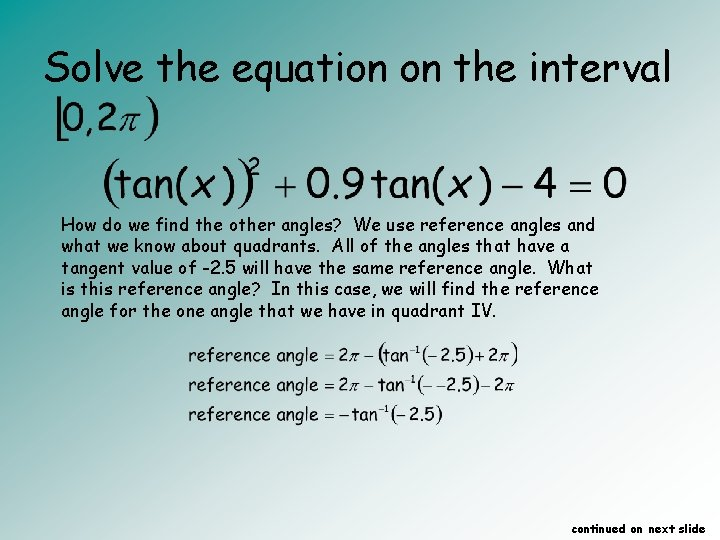Solve the equation on the interval How do we find the other angles? We use reference angles and what we know about quadrants. All of the angles that have a tangent value of -2. 5 will have the same reference angle. What is this reference angle? In this case, we will find the reference angle for the one angle that we have in quadrant IV. continued on next slide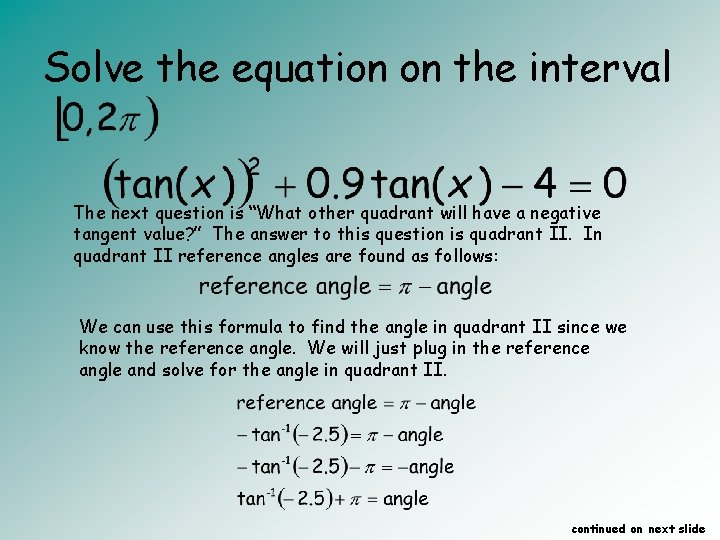Solve the equation on the interval The next question is “What other quadrant will have a negative tangent value? ” The answer to this question is quadrant II. In quadrant II reference angles are found as follows: We can use this formula to find the angle in quadrant II since we know the reference angle. We will just plug in the reference angle and solve for the angle in quadrant II. continued on next slide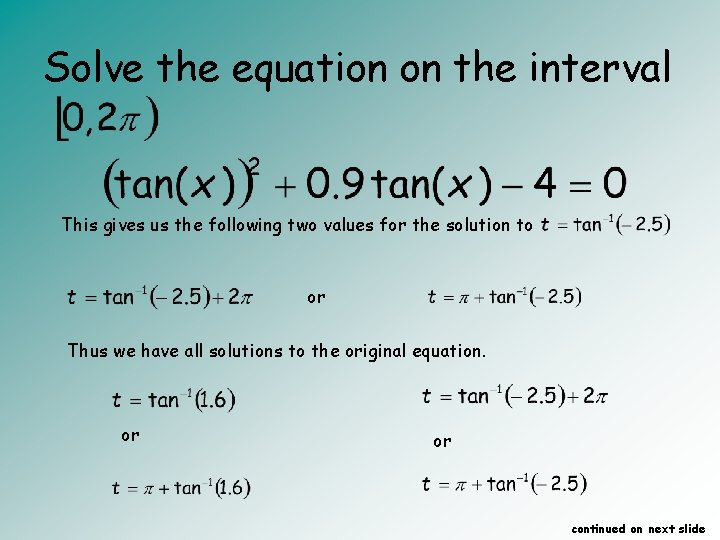Solve the equation on the interval This gives us the following two values for the solution to or Thus we have all solutions to the original equation. or or continued on next slide